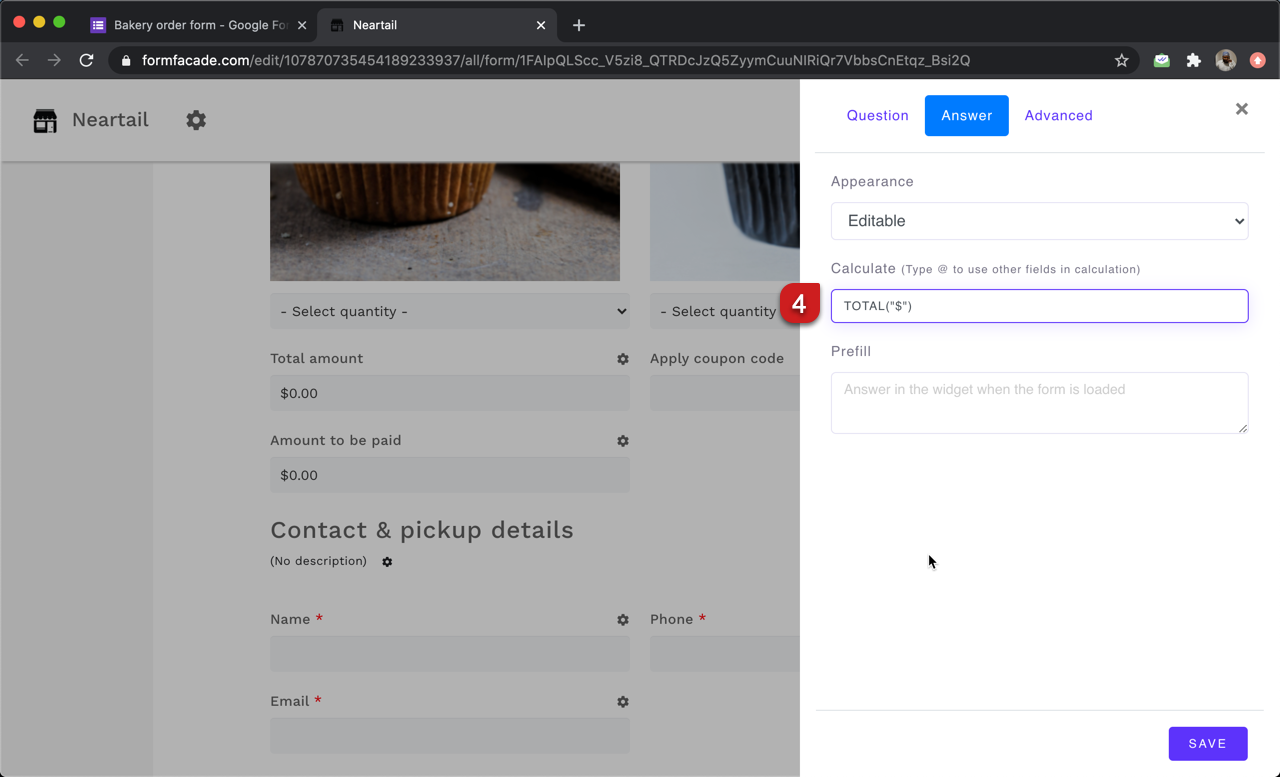### You can use the TOTAL function to calculate the total amount for the products ordered by the user and display it in the form in real-time.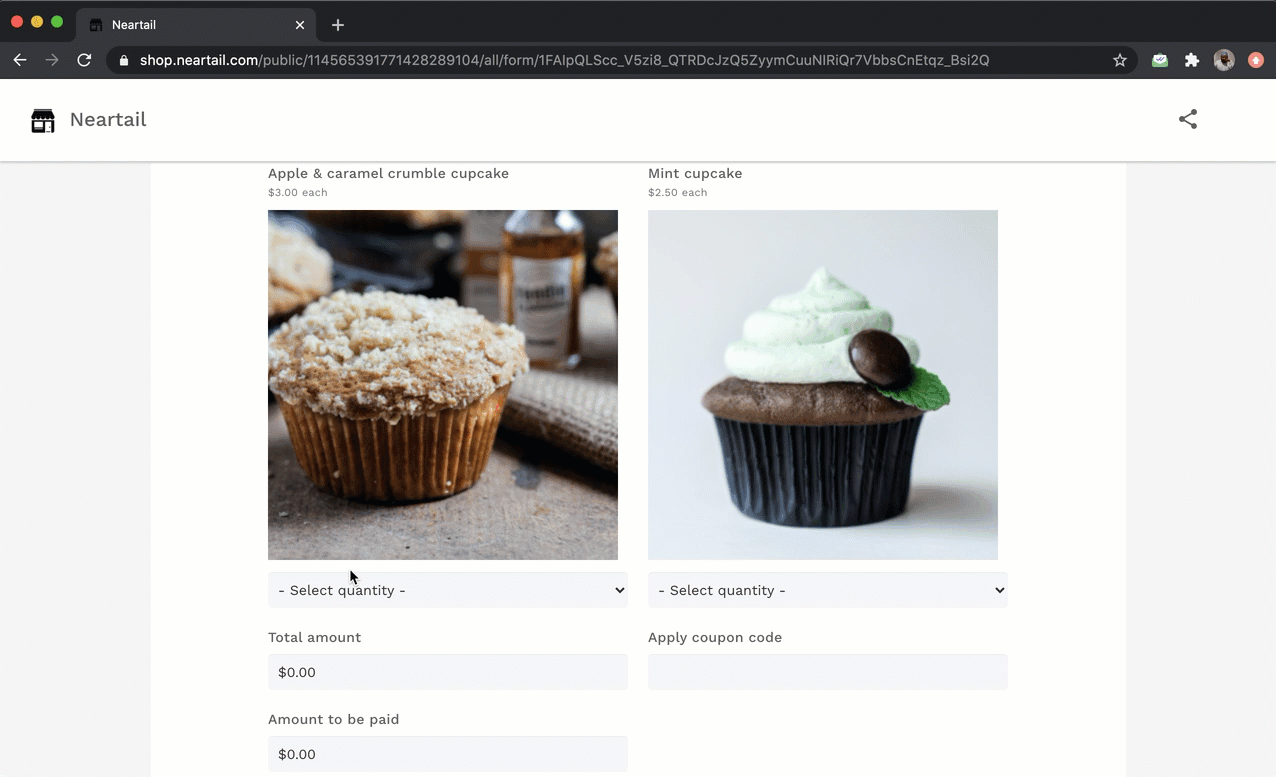#### How to use the TOTAL function to calculate the total amount?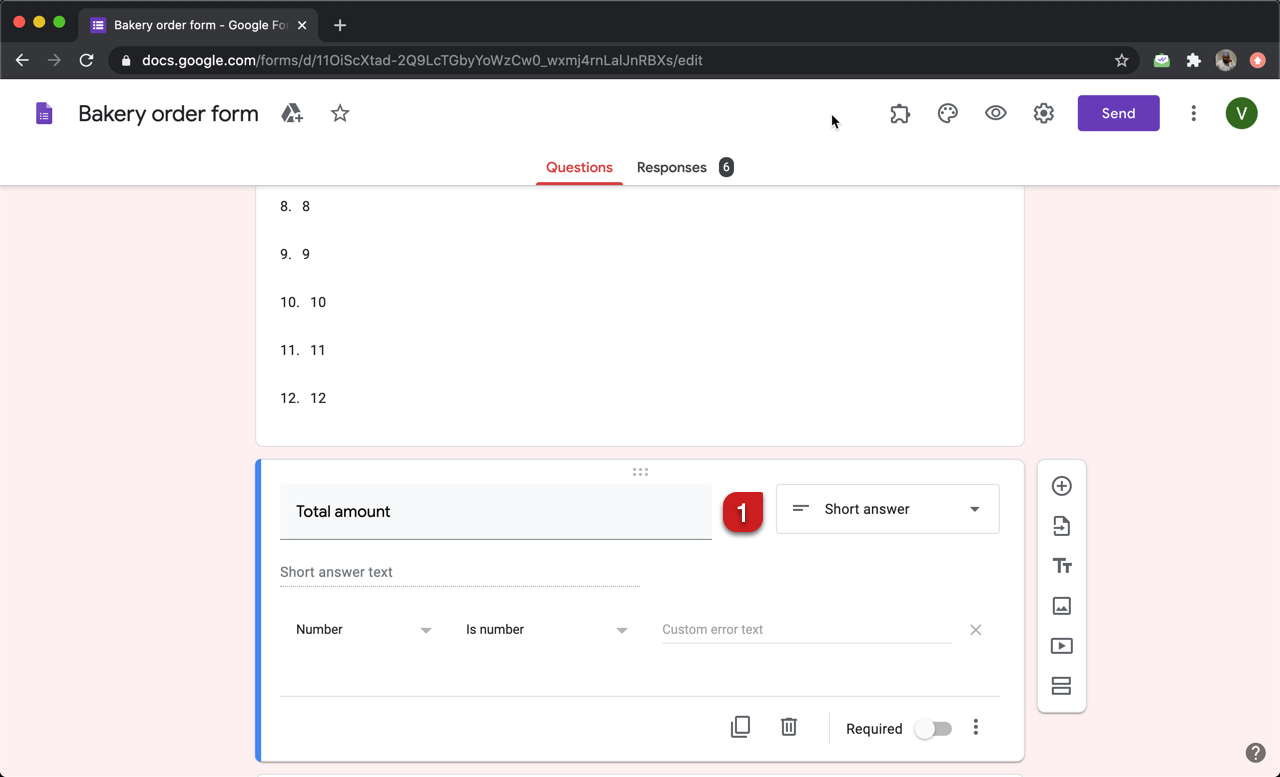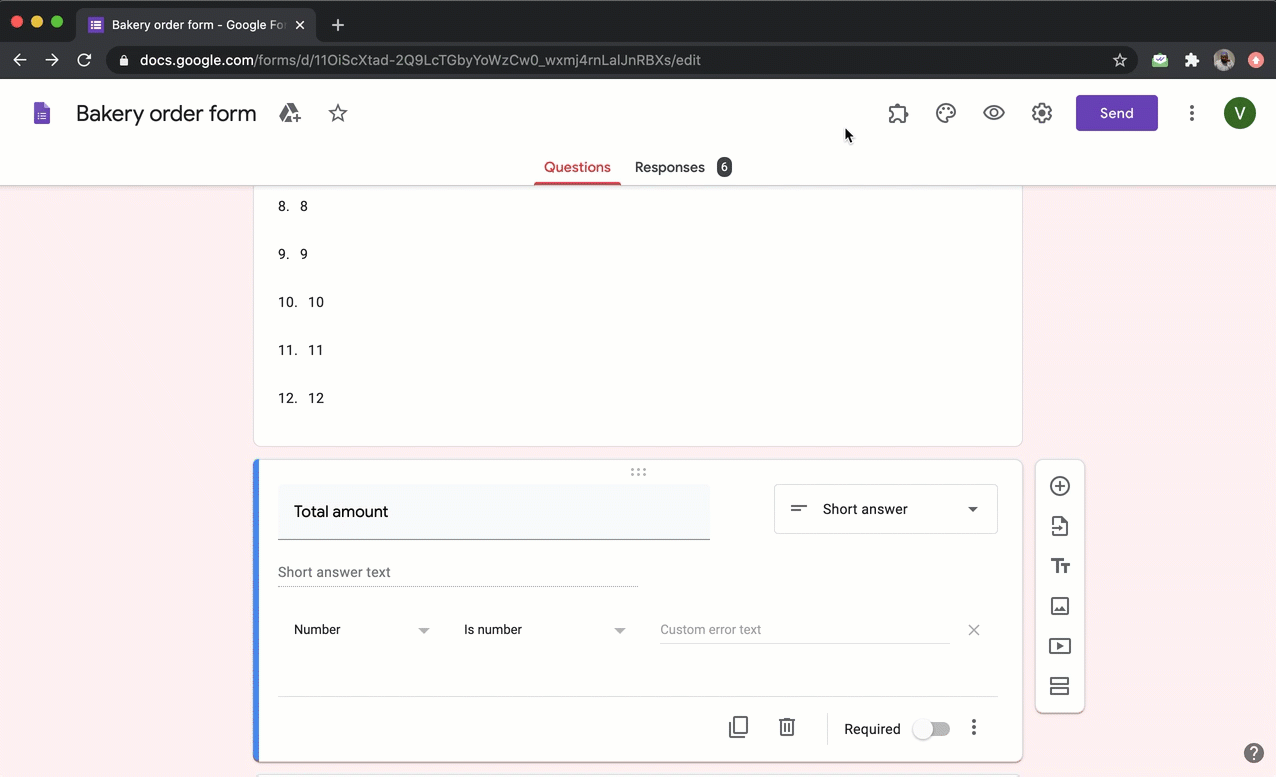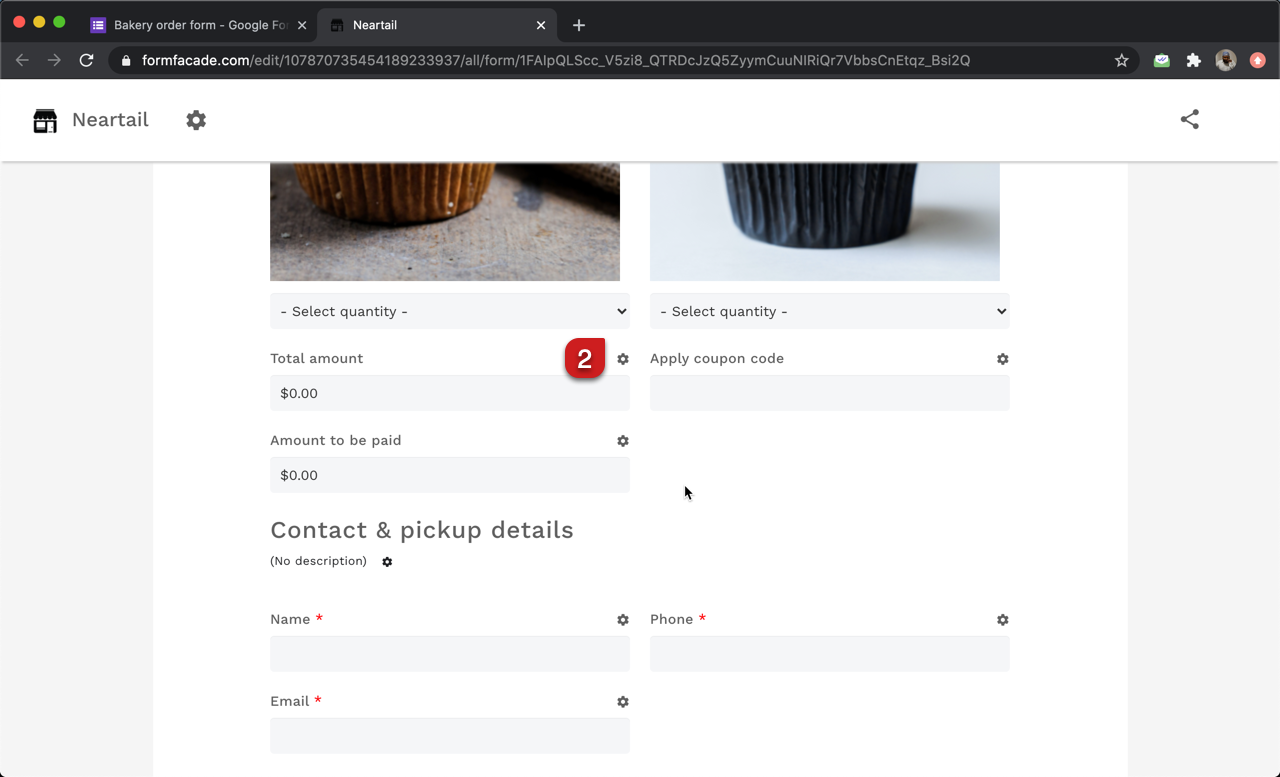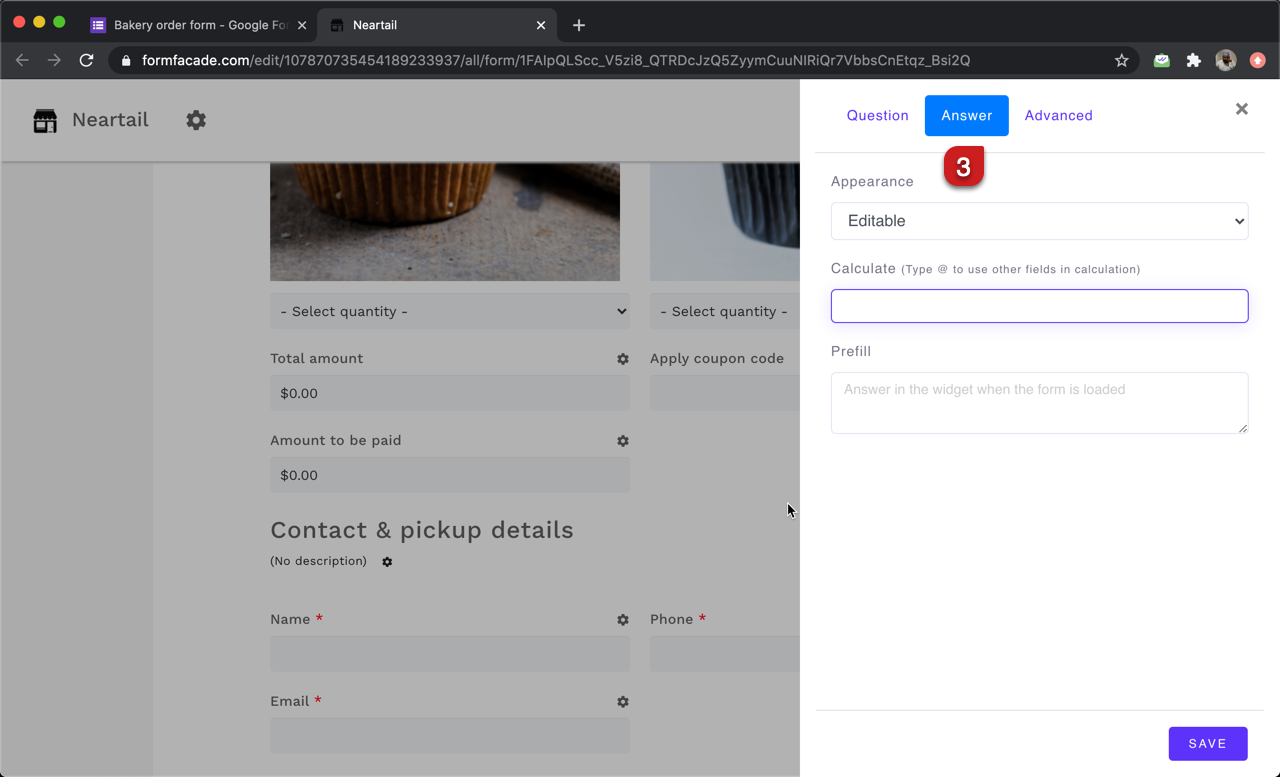#### TOTAL function

You can use the TOTAL function to calculate the total amount for the products ordered. The syntax for the TOTAL function is:

TOTAL(currency)
where

• currency = currency identifier to find the price in the description for all question types/answer choices for multiple choice, checkbox and grid questions. It can be a symbol or text, but it has to exactly match the format used in the description/answer choices.

For example:

• If the price format used in description is \$50 each, \$5.75 per kilo etc, the formula to calculate the total amount is TOTAL("\$")
• If the price format used in description is €50 each, €5.75 per kilo etc, the formula to calculate the total amount is TOTAL("€")